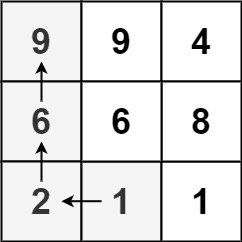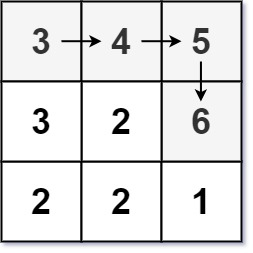### LeetCode• ㊗️
• 大家
• offer
• 多多！

## Problem

Given an m x n integers matrix, return the length of the longest increasing path in matrix.

From each cell, you can either move in four directions: left, right, up, or down. You may not move diagonally or move outside the boundary (i.e., wrap-around is not allowed).

Example 1:Input: matrix = [[9,9,4],[6,6,8],[2,1,1]]
Output: 4
Explanation: The longest increasing path is [1, 2, 6, 9].


Example 2:Input: matrix = [[3,4,5],[3,2,6],[2,2,1]]
Output: 4
Explanation: The longest increasing path is [3, 4, 5, 6]. Moving diagonally is not allowed.


Example 3:

Input: matrix = []
Output: 1


## Code

class Solution {
public int longestIncreasingPath(int[][] matrix) {
if(matrix == null | matrix.length == 0) return 0;
int m = matrix.length;
int n = matrix.length;

int[][] memo = new int[m][n];
int res = 1;

for(int i = 0; i < m; i++){
for(int j = 0; j < n; j++){
res = Math.max(res, helper(matrix, i, j, memo, new boolean[m][n], Integer.MIN_VALUE));
}
}

return res;
}

private int helper(int[][] matrix, int i, int j, int[][] memo, boolean[][] visited, int prev){
if(i < 0 || i >= matrix.length || j < 0 || j >= matrix.length || visited[i][j] || prev >= matrix[i][j]) return 0;
// visited[i][j] = true;
if(memo[i][j] != 0) return memo[i][j];

int up = helper(matrix, i - 1, j, memo, visited, matrix[i][j]) + 1;
int down = helper(matrix, i + 1, j, memo, visited, matrix[i][j]) + 1;
int left = helper(matrix, i, j - 1, memo, visited, matrix[i][j]) + 1;
int right = helper(matrix, i, j + 1, memo, visited, matrix[i][j]) + 1;
visited[i][j] = false;

memo[i][j] = Math.max(up, Math.max(down, Math.max(left, right)));
return memo[i][j];
}
}